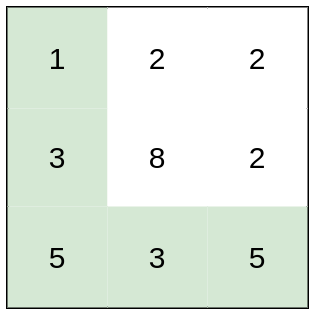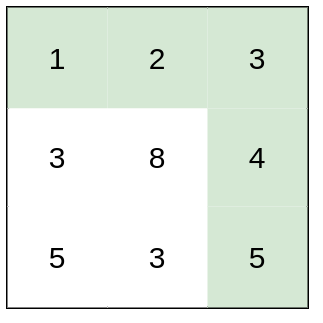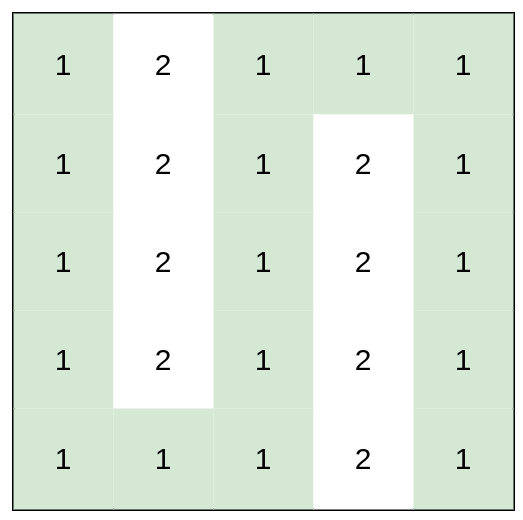# LeetCode 1631. Path With Minimum Effort Solution

You are a hiker preparing for an upcoming hike. You are given heights, a 2D array of size rows x columns, where heights[row][col] represents the height of cell (row, col). You are situated in the top-left cell, (0, 0), and you hope to travel to the bottom-right cell, (rows-1, columns-1) (i.e., 0-indexed). You can move up, down, left, or right, and you wish to find a route that requires the minimum effort.

A route's effort is the maximum absolute difference in heights between two consecutive cells of the route.

Return the minimum effort required to travel from the top-left cell to the bottom-right cell.

Example 1:Input: heights = [[1,2,2],[3,8,2],[5,3,5]]
Output: $2$
Explanation: The route of [1,3,5,3,5] has a maximum absolute difference of $2$ in consecutive cells. This is better than the route of [1,2,2,2,5], where the maximum absolute difference is $3$.

Example 2:Input: heights = [[1,2,3],[3,8,4],[5,3,5]]
Output: $1$
Explanation: The route of [1,2,3,4,5] has a maximum absolute difference of $1$ in consecutive cells, which is better than route [1,3,5,3,5].

Example 3:Input: heights = [[1,2,1,1,1],[1,2,1,2,1],[1,2,1,2,1],[1,2,1,2,1],[1,1,1,2,1]]
Output: $0$
Explanation: This route does not require any effort.

Constraints:

rows == heights.length
columns == heights[i].length
$1 \leq \text{rows}, \text{columns} \leq 100$
$1 \leq$ heights[i][j] $\leq 10^6$

## Brute Force

Our most simple brute force for this problem would be to try all different routes that start from the top-left cell and end in the bottom-right cell. We'll then find the efforts for all these routes and return the smallest.

## Full Solution

Instead of thinking of finding the efforts of all possible routes, we should think about finding routes that have some specific effort. Given some effort $a$, how do we check if there exists a route that has an effort smaller or equal to $a$?

Let's first denote the distance between two adjacent cells as the absolute difference between their heights.

A route will have an effort smaller or equal to $a$ if it travels from the top-left cell to the bottom-right cell and all adjacent cells in the route have a distance that doesn't exceed $a$. To check if such routes exist, we can check if there exists a path from the top-left cell to the bottom-right cell such that we never travel between cells with a distance that exceeds $a$. We can accomplish this with a BFS/flood fill algorithm.

We'll denote an effort $a$ as good if there exists a route with an effort smaller or equal to $a$.

The minimum effort $a$ that's good is the final answer that we return. To find the minimum effort, we can implement a binary search. Why can we binary search this value? Let's say our minimum good effort is $b$. Our binary search condition is satisfied since all values strictly less than $b$ are not good and all values greater or equal to $b$ are good.

Every binary search iteration, we can check whether or not some effort is good by running the BFS/flood fill algorithm mentioned above.

### Time Complexity

Let's denote $R$ as number of rows, $C$ as number of colmns, and $M$ as maximum height in heights.

Since BFS/flood fill will run in $\mathcal{O}(RC)$ and we have $\mathcal{O}(\log{M})$ binary search iterations, our final time complexity will be $\mathcal{O}(RC\log{M})$.

Time Complexity: $\mathcal{O}(RC\log{M})$

## C++ Solution

1class Solution {
2    const vector<int> deltaRow = {-1, 0, 1, 0};
3    const vector<int> deltaCol = {0, 1, 0, -1};
4    bool isValidEffort(vector<vector<int>>& heights, int mid) {
5        int rows = heights.size();
6        int columns = heights.size();                        // dimensions for heights
7        vector<vector<bool>> vis(rows, vector<bool>(columns));  // keeps track of whether or not we visited a node
8        queue<int> qRow;
9        queue<int> qCol;
10        qRow.push(0);
11        qCol.push(0);  // BFS starts in top-left cell
12        //We can also use queue<pair<int,int>> to store both the row & col in one queue
13        vis = true;
14        while (!qRow.empty()) {
15            int curRow = qRow.front();
16            qRow.pop();
17            int curCol = qCol.front();
18            qCol.pop();
19            for (int dir = 0; dir < 4; dir++) {
20                int newRow = curRow + deltaRow[dir];
21                int newCol = curCol + deltaCol[dir];
22                if (newRow < 0 || newRow >= rows || newCol < 0 || newCol >= columns) {  // check if cell is in boundary
23                    continue;
24                }
25                if (vis[newRow][newCol]) {  // check if cell has been visited
26                    continue;
27                }
28                if (abs(heights[newRow][newCol] - heights[curRow][curCol]) > mid) {  // check if distance exceeds limit
29                    continue;
30                }
31                vis[newRow][newCol] = true;
32                qRow.push(newRow);
33                qCol.push(newCol);
34                // process next node
35            }
36        }
37        return vis[rows - 1][columns - 1];
38    }
39
40   public:
41    int minimumEffortPath(vector<vector<int>>& heights) {
42        int low = -1;         // every effort less or equal to low will never be good
43        int high = (int)1e6;  // every effort greater or equal to high will always be good
44        int mid = (low + high) / 2;
45        while (low + 1 < high) {
46            if (isValidEffort(heights, mid)) {
47                high = mid;
48            } else {
49                low = mid;
50            }
51            mid = (low + high) / 2;
52        }
53        return high;
54    }
55};

## Java Solution

1class Solution {
2    final int[] deltaRow = {-1, 0, 1, 0};
3    final int[] deltaCol = {0, 1, 0, -1};
4    private boolean isValidEffort(int[][] heights, int mid){
5        int rows = heights.length;
6        int columns = heights.length; // dimensions for heights
7        boolean[][] vis = new boolean[rows][columns]; // keeps track of whether or not we visited a node
8        Queue<Integer> qRow = new LinkedList();
9        Queue<Integer> qCol = new LinkedList();
11        qCol.add(0); // BFS starts in top-left cell
12        vis = true;
13        while (!qRow.isEmpty()) {
14            int curRow = qRow.poll();
15            int curCol = qCol.poll();
16            for (int dir = 0; dir < 4; dir++) {
17                int newRow = curRow + deltaRow[dir];
18                int newCol = curCol + deltaCol[dir];
19                if (newRow < 0 || newRow >= rows || newCol < 0
20                    || newCol >= columns) { // check if cell is in boundary
21                    continue;
22                }
23                if (vis[newRow][newCol]) { // check if cell has been visited
24                    continue;
25                }
26                if (Math.abs(heights[newRow][newCol] - heights[curRow][curCol]) > mid){
27                    // check if distance exceeds limit
28                    continue;
29                }
30                vis[newRow][newCol] = true;
33                // process next node
34            }
35        }
36        return vis[rows-1][columns-1];
37    }
38    public int minimumEffortPath(int[][] heights) {
39        int rows = heights.length;
40        int columns = heights.length; // dimensions for heights
41        int low = -1; // every effort less or equal to low will never be good
42        int high = (int) 1e6; // every effort greater or equal to high will always be good
43        int mid = (low + high) / 2;
44        while (low + 1 < high) {
45            if (isValidEffort(heights,mid)) {
46                high = mid;
47            } else {
48                low = mid;
49            }
50            mid = (low + high) / 2;
51        }
52        return high;
53    }
54}

## Python Solution

1import collections
2class Solution:
3    def minimumEffortPath(self, heights: List[List[int]]) -> int:
4        rows = len(heights)
5        columns = len(heights)  # dimensions for heights
6        low = -1  # every effort less or equal to low will never be good
7        high = 10 ** 6  # every effort greater or equal to high will always be good
8        mid = (low + high) // 2
9        def isValidEffort(heights, mid):
10            vis = [[False] * columns for a in range(rows)]
11            # keeps track of whether or not we visited a node
12            qRow = collections.deque()
13            qCol = collections.deque()  # BFS starts in top-left cell
14            vis = True
15            while qRow:
16                curRow = qRow.popleft()
17                curCol = qCol.popleft()
18                for [deltaRow, deltaCol] in [(-1, 0), (0, 1), (1, 0), (0, -1)]:
19                    newRow = curRow + deltaRow
20                    newCol = curCol + deltaCol
21                    if (newRow < 0 or newRow >= rows or newCol < 0 or newCol >= columns):
22                        # check if cell is in boundary
23                        continue
24                    if vis[newRow][newCol] == True:  # check if cell has been visited
25                        continue
26                    if (abs(heights[newRow][newCol] - heights[curRow][curCol]) > mid):
27                        # check if distance exceeds limit
28                        continue
29                    vis[newRow][newCol] = True
30                    qRow.append(newRow)
31                    qCol.append(newCol)
32                    # process next node
33            return vis[rows-1][columns-1]
34        while low + 1 < high:
35            if isValidEffort(heights,mid):
36                high = mid
37            else:
38                low = mid
39            mid = (low + high) // 2
40        return high
41